Condition for Vectors to be Linearly Independent

A set of vectors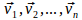is said to be linearly independent if none of the vectors can be expressed in terms of the others, so that no constants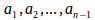exist so that fr example,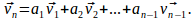This is equivalents to there being no constants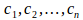satisying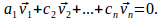If the set of vectors is not linearly independent, then it is linearly dependent.

In a space of dimension m, so that each vector has m components, the set of vectorswill be linearly dependent if n&gt;m.

If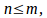the set of vectors may or may not be linearly independent.

If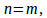we can write the set of vectors as as square matrix. The vectors will then be linearly dependent if and only if the determinant of the matrix is zero. This is because one of the columns is a linear combination of the other columns, so column reduction would result in a column of zeros. Expanding along that columm would return a zero determinant. If the determinant is not zero, the set of vectors is linearly independent.

Example: Determine whether or not the vectors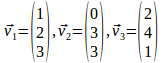are linearly independent.

Writing the vectors as the columns of a matrix gives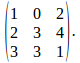Then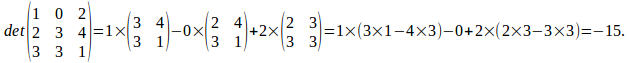The vectors are linearly independent.